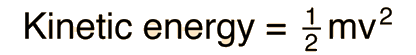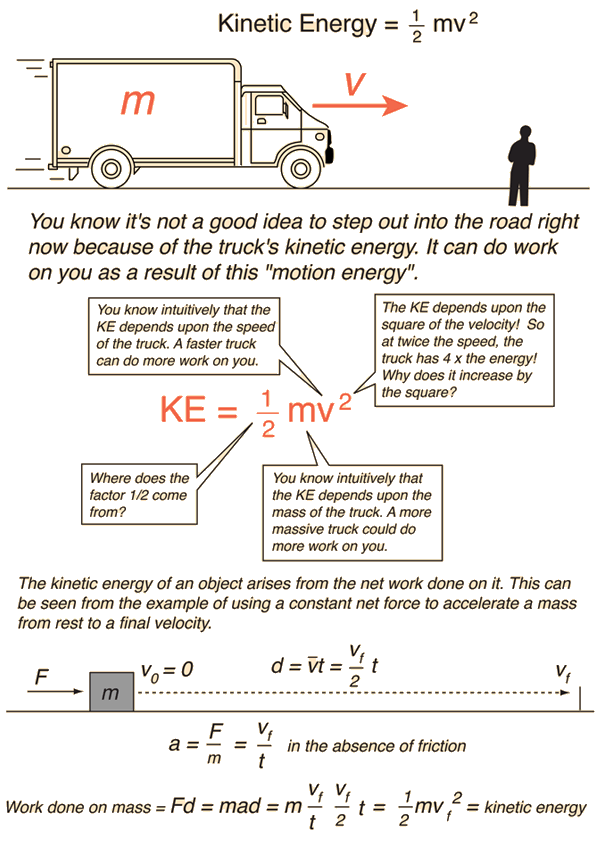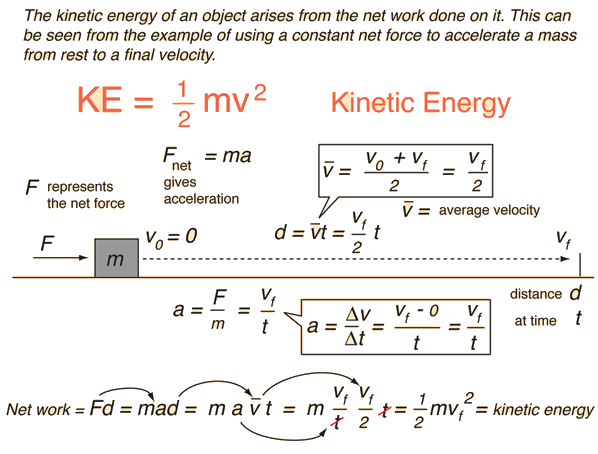# Kinetic Energy

Kinetic energy is energy of motion. The SI unit for energy is the joule = newton x meter in accordance with the basic definition of energy as the capacity for doing work. The kinetic energy of an object is the energy it possesses because of its motion. The kinetic energy* of a point mass m is given byKinetic energy is an expression of the fact that a moving object can do work on anything it hits; it quantifies the amount of work the object could do as a result of its motion. The total mechanical energy of an object is the sum of its kinetic energy and potential energy.The total energy of an isolated system is subject to the conservation of energy principle.

For an object of finite size, this kinetic energy is called the translational kinetic energy of the mass to distinguish it from any rotational kinetic energy it might possess - the total kinetic energy of a mass can be expressed as the sum of the translational kinetic energy of its center of mass plus the kinetic energy of rotation about its center of mass.

*This assumes that the speed is much less than the speed of light. If the speed is comparable with c then the relativistic kinetic energy expression must be used

 Development of the kinetic energy concept
Index

Energy concepts

 HyperPhysics***** Mechanics R Nave
Go Back

# Kinetic Energy Concept

Kinetic energy is energy of motion. The kinetic energy of an object is the energy it possesses because of its motion. The kinetic energy of a point mass m is given byMore detail on this development

Energy as the capacity for doing work is a convertible currency. To give something kinetic energy you must do work on it. This development uses the concept of work as well as Newton's second law and the motion equations. It is a special case of the work-energy principle, a powerful general principle of nature.

Index

Energy concepts

 HyperPhysics***** Mechanics R Nave
Go Back

# More Detail on Kinetic Energy Concept

Kinetic energy is energy of motion. The kinetic energy of an object is the energy it possesses because of its motion. The kinetic energy of a point mass m is given byConcepts involved in relating net work to change in kinetic energy:

 Newton's 2nd Law: F=ma Acceleration Average velocity Work-energy principle Work Motion equations
Index

Energy concepts

 HyperPhysics***** Mechanics R Nave
Go Back